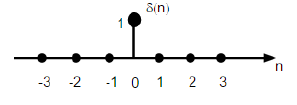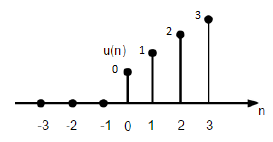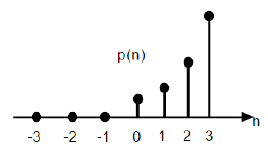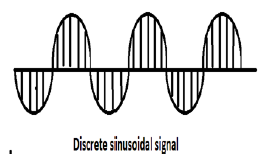# Digital Signal Processing - Basic DT Signals

#### Digital Marketing Online Training

23 Lectures 2 hours

#### How To Start Your Own Digital Marketing Agency

125 Lectures 9 hours

#### Digital Marketing Agency Elite Consultants Masterclass

140 Lectures 20 hours

We have seen that how the basic signals can be represented in Continuous time domain. Let us see how the basic signals can be represented in Discrete Time Domain.

## Unit Impulse Sequence

It is denoted as δ(n) in discrete time domain and can be defined as;

$$\delta(n)=\begin{cases}1, & for \quad n=0\\0, & Otherwise\end{cases}$$## Unit Step Signal

Discrete time unit step signal is defined as;

$$U(n)=\begin{cases}1, & for \quad n\geq0\\0, & for \quad n<0\end{cases}$$The figure above shows the graphical representation of a discrete step function.

## Unit Ramp Function

A discrete unit ramp function can be defined as −

$$r(n)=\begin{cases}n, & for \quad n\geq0\\0, & for \quad n<0\end{cases}$$The figure given above shows the graphical representation of a discrete ramp signal.

## Parabolic Function

Discrete unit parabolic function is denoted as p(n) and can be defined as;

$$p(n) = \begin{cases}\frac{n^{2}}{2} ,& for \quad n\geq0\\0, & for \quad n<0\end{cases}$$

In terms of unit step function it can be written as;

$$P(n) = \frac{n^{2}}{2}U(n)$$The figure given above shows the graphical representation of a parabolic sequence.

## Sinusoidal Signal

All continuous-time signals are periodic. The discrete-time sinusoidal sequences may or may not be periodic. They depend on the value of ω. For a discrete time signal to be periodic, the angular frequency ω must be a rational multiple of 2π.A discrete sinusoidal signal is shown in the figure above.

Discrete form of a sinusoidal signal can be represented in the format −

$$x(n) = A\sin(\omega n + \phi)$$

Here A,ω and φ have their usual meaning and n is the integer. Time period of the discrete sinusoidal signal is given by −

$$N =\frac{2\pi m}{\omega}$$

Where, N and m are integers.